## Poor Use of Mathematical Language (PUML)

A prerequisite skill to writing good mathematics is the ability to write well in one's native tongue. People who cannot write a complete English sentence should take remediation in English composition before reading on.

What may surprise some students is that good writing using mathematical symbols (even in the write-up of homework problems) consists of using complete sentences, setting up one's ideas clearly and then following through on the details, much as one expects from a good English essay. The language and symbols of mathematics are used just like regular English words and phrases to express ideas, albeit ideas which one would often struggle to use any other means of expressing.

Nobody studies mathematical writing as a subject. Your mathematics professor(s) got to be good writers of mathematics, if good they be, by reading papers and books by other mathematicians, not by reading a treatise such as this one. If a book on good mathematical writing does exist (and there are probably a number of such books), they will say much more than I say here. I will only describe the most common example of poor mathematical writing I see when grading students' work: Using Equals as a Conjunction (UEC).

The word equals" has a very specific meaning. It requires two objects, and it asserts that these two objects are the same. In mathematics, the two objects are usually quantities, like the mathematical expression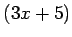, or the number 7. Even within this tight definition, mathematical equations, as I mentioned earlier, come in two varieties: identities and conditional equations. A conditional equation is one such as the equation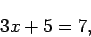which is only for particular values of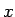(in this case one particular value). In algebra courses one often sees conditional equalities in homework problems accompanied by the instruction Solve the equation". There are some quantities that are the same regardless of the value of the variable. A familiar example is the identity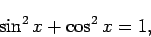which is true no matter what real valuetakes. These two types of equations encompass the two most common (and only?) valid ways to use an equals' (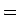) sign.

Consider the typical calculus problem of evaluating a limit like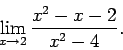What we are given here is not an equation, but an expression. If we begin writing a series of equalities to simplify/evaluate this expression, we will want them to be identities, as in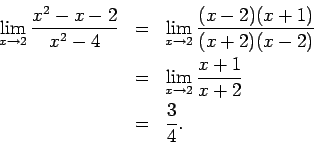The original expression, along with each of the ensuing expressions, as it turns out, are all equal to the number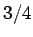.

In contrast, suppose we begin with a (conditional) equation likewhich we are asked to solve. If a student who understands very well the discussion of UAS and UMD (found earlier in this piece) makes a mistake, she is most likely to do so writing something like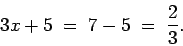UEC

Such a string of equalities asserts three things:
1. that,
2. that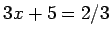,
3. and that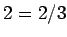.
(i) and (ii) are conditional equations in their own right, but it should be clear that they do not have the same solutions as the original equation(nor does (i) have the same solution as (ii)). And (iii) has no solution at all, for it is never true. What I am really saying is that the string of equations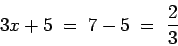(1)

is really three equations, and there is no common solution between them (and, even if there had been, such a solution would have no relevance to the original problem, that of solving). The student most likely never intended to assert these three equations in place of the original; she simply began writing out her ideas, and used an equals sign to join them together whenever it seemed some sort of conjunction was required.

The student who writes (1) actually appears to have some facility in the techniques for solving linear equations, but lacks the ability to put her ideas onto paper in a meaningful fashion. One good way to express the solution of the previous equation is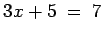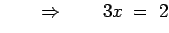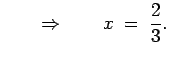(2)

The symbol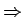can be translated here as which implies". Yes, (2) is more writing than (1), much in the same way the complete sentence I am taking the train to Chicago this weekend" requires more writing than the three words `weekend, Chicago, train". A more favorable comparison is between (2) and the same ideas expressed in English words: If the sum of three timesand five is seven, then subtracting five from both sides and dividing by three yields the value of two-thirds for, or, perhaps more literally, Assuming that the sum of three timesand five is seven, this implies that three timesis two, and thatis two-thirds.

Top Algebra Errors Made by Calculus Students (full document)